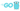# Go - Learning 'for' loop statement in-depth

10 Aug, 2021, 4 min read

## Go - Learning 'for' loop statement in-depth

In programming, repeating a particular task is essential. Loop helps in executing pieces of code multiple times based on a particular condition. Let's learn about how you can use the loop in Go to your advantage.

### What is For loop

`for` is the statement available in Go for repeating block of code. There is a loop condition provided for the statement and the block of code executes until the condition turns false.

``````for initialize; condition; after_loop {

}``````

Each execution of the loop is called `iteration`

There are three parts to the `for` loop

• initialize - Runs only once at the start of the loop
• condition - Check every time before each iteration
• after_loop - Statement runs after each iteration is complete

### Example - Printing from 1 to 10 using loop

``````package main

import "fmt"

func main() {

for i:= 1; i <= 10; i++ {
fmt.Println(i)
}

}``````

Loop execution starts with initializing the value of `i`. This will be set to 1 as per our example above. Then the loop checks the condition `i <= 10`. The condition becomes true and so the loop executes the code inside the block. After the execution of the block, it executes the post statement `i++`. Value of i is incremented and then the new value is set will be `1`. Now the condition check happens again and the cycle continues till the condition becomes false.

Output:

``````1
2
3
4
5
6
7
8
9
10
``````

### Example - Practical counter example

Consider the scenario where you are creating a student management application. All student's total marks are present inside one array. One of the requirements is to print the total number of students who have scored more than 70.

``````package main

import "fmt"

func main() {
total_marks:= [...]int{46, 54, 23, 64, 74, 93, 64, 79}

student_above70 := 0
for i:=0; i < 8; i++ {
if total_marks[i] > 70 {
student_above70 ++
}
}

fmt.Println(student_above70)
}``````

### Break statement

Some scenarios require our code execution inside the loop to stop when we have some condition. This can be achieved using the `break` statement.

``````package main

import "fmt"

func main() {

for i:= 1; i <= 5; i++ {
if i % 3 == 0 {
break;
}
fmt.Printf("%d ", i)
}

}``````

The above program runs the loop from 1 to 5 and prints its value. We also have a special condition to check if the number is divisible by 3. If that is the case, we are using the `break` statement to stop the execution of the loop and move to the next line after the loop

This code output will be the following

``1 2 ``

### Continue Statement

`Continue` is used to skip the execution of the code in the current iteration and move the control to the next iteration of the loop. Statement after the continue will not be executed.

``````package main

import "fmt"

func main() {

for i:= 1; i <= 5; i++ {
if i % 3 == 0 {
continue
}
fmt.Printf("%d ", i)
}
``````

Let's have a look at a similar example as before. We are adding the continue statement for all the numbers which are divisible by 3. Since the print statement is after the continue statement, it will skip the print for the numbers which meet the condition

``````Output:

1 2 4 5 ``````

### Infinite loop

It is possible to create an infinite loop in Go without providing a condition in the `for` loop. Programmers dread the infinite loop but it can be useful when you don't want your program to close and you are waiting for something.

``````package main

import "fmt"

func main() {
for {
fmt.Println("Infinite Loop!")
}
}``````

### Mulitple initialization in for loop

Go allows adding more than one initialization variable. This can be helpful if you are using those variables inside the loop

``````
package main

import "fmt"

func main() {

for start, end := 1, 10 ; start <= end; start, end = start + 1, end - 1{
fmt.Printf("%d ", start)
}

}``````

Multiple assignments is achieved by separating the variables on one side and value on the other side using `:=` operator and comma in between the variables.

``a, b, c := 0, 1, 2``

Each of the variables takes the respective values from the other side. so `a = 0`, `b = 1`, `c = 2`

## Practical Assignment - Try these out

1. Build an application that prints out the average of the student's score
2. Build an application that will find the sum of all numbers divisible by 4 till 100

Use the live code editor below to get started on the assignments

## Summary

Loop forms an essential part of any application and it is a great asset to the programmer's toolkit. Practice these assignments to get a better understanding of the loop and excel in your programming tasks.

## Conclusion

Stay tuned by subscribing to our mailing list and joining our Discord community

Discord